# Linux expr command tutorial for beginners (with examples)

Sometimes, while working on the command line (especially when dealing with a shell script), you may find yourself in a situation where you have to perform actions like searching for a substring in a string, finding its index, as well as other things like performing comparisons and arithmetic operations.

For those who aren't in the know, there exists a command line utility - dubbed expr - that lets you do all this. In this tutorial, we will discuss the basics of this command along with some of the features it provides. Please note that all examples and instructions mentioned here have been tested on Ubuntu 16.04LTS.

## Linux expr command

The tool's official documentation says it's used to evaluate expressions. Here's is the syntax of the expr command:

``expr EXPRESSIONexpr OPTION``

Following are some Q&A-style examples that'll give you a good idea about how this tool works.

## Q1. How to perform arithmetic operations using expr?

Suppose you want to add two numbers (say, 5 and 6), here's how you can do this using expr:

``expr 5 + 6``

Here's the above command in action: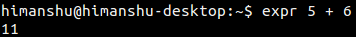So you can see that the sum '11' was produced in the output. Please note that a single space on either side of the operator ('+' in this case) is mandatory. Otherwise, the expr command would produce the following output: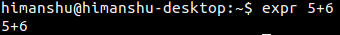which is clearly wrong, and not what we expect.

Similarly, a subtraction operation can be carried out in the following way:

``expr 15 - 6``

Here's an example of division:

``expr 10 / 3``

Note that the aforementioned command will only produce 3 in output. In case you want to see the remainder, use the % operator.

``expr 10 % 3``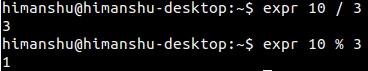Multiplying should ideally be achieved in the following way:

``expr 10 * 3``

But this doesn't work, because * is a built-in shell operator as well. So, in order to make it behave like a multiplication operator, you need to precede it with an escape character in the following way:

``expre 10 \* 3``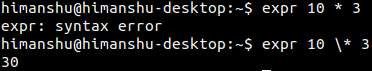## Q2. How to perform comparison operations using expr?

The expr command also lets you perform comparison operations in various ways. For example, the following command (third in the screenshot below) will return ARG1 if it is neither null nor 0, otherwise ARG2.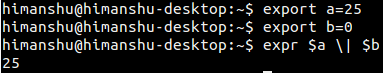Please note that the backslash before pipe is used as an escape character as pipe is otherwise treated as a built-in shell operator.

Similarly, you can perform a lot of operations. Following screenshot - taken from the command's man page - should give you a good idea regarding what kind of comparisons you can do with the expr command.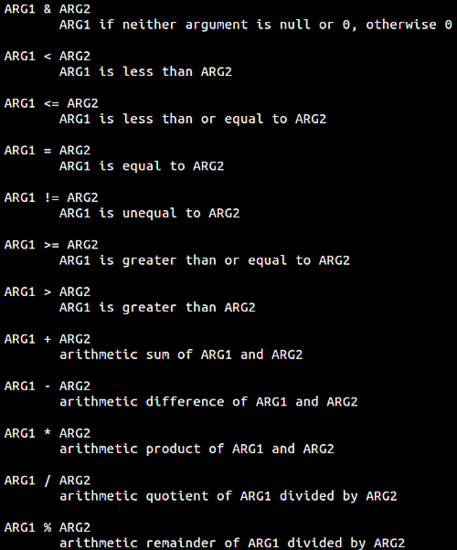## Q3. How to perform string-related operations using expr?

The expr command also lets you perform several string-related operations. For example, to find length of a string, you can use the tool in the following way:

``expr length [string]``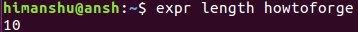In case the string contains spaces, you need to use the escape character.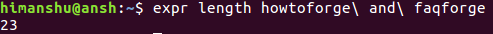Moving on, you can also use expr to extract a substring in a given string. Here's the syntax:

expr substr [string] [pos] [length]

For example, to fetch 'forge' from 'howtoforge', you  can use the tool in the following way: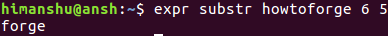What's more, you can also use expr to find starting index for a set of characters in a given string. Here's the syntax for it

expr index [string] [chars]

For example, to find the index of 'wt' in 'howtoforge', use expr in the following way: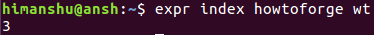## Conclusion

As you'll likely agree, expr is a feature-rich command, but yet easy to understand and simple to use. Here, in this tutorial, we've tried to provide you information regarding most of the basic features it offers. Once you're done practicing these, you can head to the tool's man page to learn more about it.view as pdf |print

## 1 Comment(s)

Add comment

### Comments

By: Spencer

A question I have is: does expr start counting at 0 when looking at length or does it start with one? From the given examples, they seem to indicate the latter.